Skip to main content Accessibility help
Home

### Local theory (14Bxx)

• Article
• ##### Ascending chain condition for$F$ -pure thresholds on a fixed strongly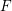$F$ -regular germ
• Compositio Mathematica, Volume 155, Issue 6

### Cycles and subschemes (14Cxx)

• Article
• ##### Log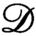$\mathscr{D}$-modules and index theorems
• Forum of Mathematics, Sigma, Volume 9

### Families, fibrations (14Dxx)

• Article
• ##### POWER SERIES PROOFS FOR LOCAL STABILITIES OF KÄHLER AND BALANCED STRUCTURES WITH MILD$\partial \overline {\partial }$-LEMMA
• Nagoya Mathematical Journal, First View

### Birational geometry (14Exx)

• Article
• ##### MINIMAL MODEL THEORY FOR LOG SURFACES IN FUJIKI’S CLASS${\mathcal{C}}$
• Nagoya Mathematical Journal, First View

### Arithmetic problems. Diophantine geometry (14Gxx)

• Article
• ##### Logarithmic growth filtrations for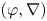$(\varphi ,\nabla )$-modules over the bounded Robba ring
• Compositio Mathematica, Volume 157, Issue 6

### Curves (14Hxx)

• Article
• ##### Pencils on Surfaces with Normal Crossings and the Kodaira Dimension of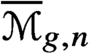$\overline {\mathcal {M}}_{g,n}$
• Forum of Mathematics, Sigma, Volume 9

### Algebraic groups (14Lxx)

• Article
• ##### Categorification via blocks of modular representations for$\mathfrak {sl}_n$
• Canadian Journal of Mathematics, First View

### Projective and enumerative geometry (14Nxx)

• Article
• ##### Holomorphic anomaly equation for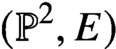$({\mathbb P}^2,E)$ and the Nekrasov-Shatashvili limit of local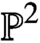${\mathbb P}^2$
• Forum of Mathematics, Pi, Volume 9

### Affine geometry (14Rxx)

• Article
• ##### ON SEPARABLE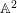$\mathbb{A}^{2}$ AND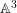$\mathbb{A}^{3}$ -FORMS
• Nagoya Mathematical Journal, Volume 239